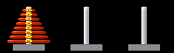Loading

# Different mathematical solutions

There are a couple of mathematical ways to solve Tower of Hanoi and we cover two of these:

• The simple algorithmic solution: Though the original puzzle featured 64 disks, according to popular belief, the game can be played with any number of rings. Mathematicians have come up with a simple algorithm that can predict the number of moves in which the game can be solved. The formula for this theory is 2n -1, with "n" being the number of rings used.
• Recursive solution: This method involves the use of the principles of mathematical induction and recurrence relations. The formula for reducing the number of moves required to solve the puzzle is , whereby "h" denotes the height of the tower of a specific number of disks, which has to be moved from one rod to another.

Above is a unique application that shows you exactly how the puzzle is solved including the number of moves. Select the number of disks and click start to see how it is resolved.

In case of a board game, there are different methods to solve it. In an hypothetical situation where there is just one disk (that is N=1) you can do the following:
1. Move it from rod 1 to rod 3
2. If there is more than one disk, ignore the bottom disk, that way the new number of disks will be N-1.
3. Solve the problem for N-1 disks, assuming rod 2 as the destination, and rod 3 as the spare rod.
4. At the end of the fourth step, there will be N-1 rings on the second rod and the largest will still be on rod 1 (which is the bottom ring that we ignored).
5. Now, the new number of disks on rod 1 is N=1.
6. Solve the problem for N=1 disk by moving it to rod 3.
7. Move the N-1 disks from rod 2 to rod 3 (assuming rod 3 as destination and rod 1 as spare).

Below you can watch a video of the solution of tower of hanoi with 10, 11 and 12 discs: